Test: Electromagnetic Waves - 1

# Test: Electromagnetic Waves - 1 - Electrical Engineering (EE)

Test Description

## 10 Questions MCQ Test Topicwise Question Bank for Electrical Engineering - Test: Electromagnetic Waves - 1

Test: Electromagnetic Waves - 1 for Electrical Engineering (EE) 2023 is part of Topicwise Question Bank for Electrical Engineering preparation. The Test: Electromagnetic Waves - 1 questions and answers have been prepared according to the Electrical Engineering (EE) exam syllabus.The Test: Electromagnetic Waves - 1 MCQs are made for Electrical Engineering (EE) 2023 Exam. Find important definitions, questions, notes, meanings, examples, exercises, MCQs and online tests for Test: Electromagnetic Waves - 1 below.
Solutions of Test: Electromagnetic Waves - 1 questions in English are available as part of our Topicwise Question Bank for Electrical Engineering for Electrical Engineering (EE) & Test: Electromagnetic Waves - 1 solutions in Hindi for Topicwise Question Bank for Electrical Engineering course. Download more important topics, notes, lectures and mock test series for Electrical Engineering (EE) Exam by signing up for free. Attempt Test: Electromagnetic Waves - 1 | 10 questions in 30 minutes | Mock test for Electrical Engineering (EE) preparation | Free important questions MCQ to study Topicwise Question Bank for Electrical Engineering for Electrical Engineering (EE) Exam | Download free PDF with solutions
 1 Crore+ students have signed up on EduRev. Have you?
Test: Electromagnetic Waves - 1 - Question 1

### Consider the following statements associated with an electromagnetic wave in free space: At every point in space, the electric field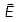and th emagnetic vector field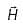are perpendicular to each other and to the direction of propagation. Velocity of propagation of wave in free space is equal to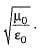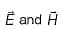oscillate in phase opposition and the ratio of their amplitude is constant being equal to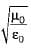Whatever may be the frequency , the electromagnetic wave travels in space with velocity of light. Which of the above statements are correct?

Detailed Solution for Test: Electromagnetic Waves - 1 - Question 1
• Velocity of propagation of EM-wave in free space is equal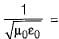= speed of light (3 x 108 m/s). Hence statement-2 is not correct.
•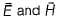oscillate in phase and the ratio of their amplitude is constant being equal to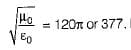. Hence, statement-3 is not correct.

Thus, statements-1 and 4 are only correct.

Test: Electromagnetic Waves - 1 - Question 2

### Intrinsic impedance or charcteristic impedance of the non-conducting medium in the free space is

Detailed Solution for Test: Electromagnetic Waves - 1 - Question 2

Intrinsic impedance or characteristic impedance of the non-conducting medium in free space is given as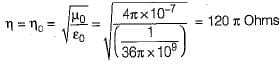Test: Electromagnetic Waves - 1 - Question 3

### If the magnitude of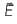in a plane wave is 1 V/m, what is the magnitude of H for a plane wave in free space?

Detailed Solution for Test: Electromagnetic Waves - 1 - Question 3

We know that,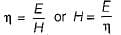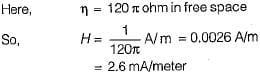Test: Electromagnetic Waves - 1 - Question 4

What is the velocity of a plane wave in a lossless medium having a relative permittivity of 5 and relative permeability of unity?

Detailed Solution for Test: Electromagnetic Waves - 1 - Question 4

Velocity of propagation is given by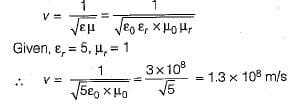Test: Electromagnetic Waves - 1 - Question 5

A plane wave in free space has an average Poynting vector of 1 Wm-2. The average energy density is

Detailed Solution for Test: Electromagnetic Waves - 1 - Question 5

Poynting vector P= EH and total energy density W= μH2.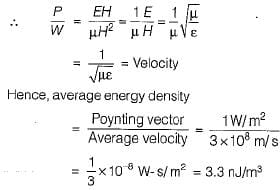Test: Electromagnetic Waves - 1 - Question 6

Infree space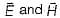are respectively given by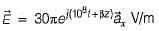and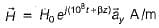The value of β and H0 are respectively

Detailed Solution for Test: Electromagnetic Waves - 1 - Question 6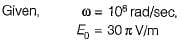This is a plane wave.
For plane wave in space,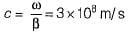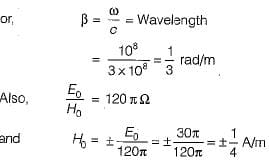Test: Electromagnetic Waves - 1 - Question 7

In a homogeneous non-conducting region where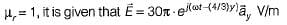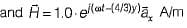The value of ∈r and ω are

Detailed Solution for Test: Electromagnetic Waves - 1 - Question 7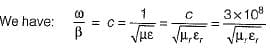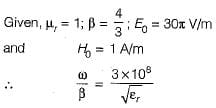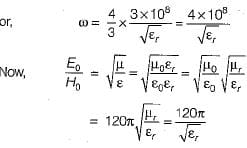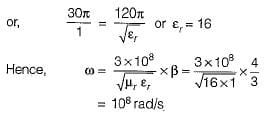Test: Electromagnetic Waves - 1 - Question 8

In free space it is given that,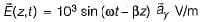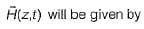Detailed Solution for Test: Electromagnetic Waves - 1 - Question 8

Looking at the phase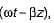we can find that direction of propagation is +z.
Since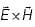must also lie in a + z direction, A must have the direction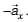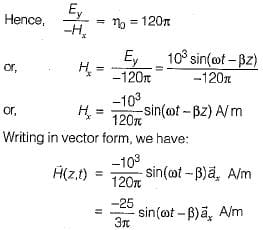Test: Electromagnetic Waves - 1 - Question 9

Assertion (A): Uniform plane electromagnetic waves are transverse in nature.
Reason (R): Uniform plane electromagnetic
waves have components of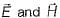only in direction perpendicular to the direction of propagation and not in the direction of propagation.

Test: Electromagnetic Waves - 1 - Question 10

Assertion (A): The term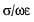is sometimes referred to the loss tangent.
Reason (R): The two vectors Jc (conduction current density) and Jd (displacement current density) are 180° out of phase and are in the opposite direction in space.

Detailed Solution for Test: Electromagnetic Waves - 1 - Question 10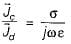which indicates that the two vectors Jc and Jd are out of phase in time and are in the same direction in the space. Hence, reason is not a correct statement.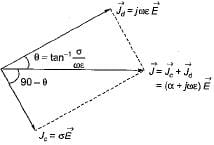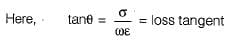## Topicwise Question Bank for Electrical Engineering

211 tests
Information about Test: Electromagnetic Waves - 1 Page
In this test you can find the Exam questions for Test: Electromagnetic Waves - 1 solved & explained in the simplest way possible. Besides giving Questions and answers for Test: Electromagnetic Waves - 1, EduRev gives you an ample number of Online tests for practice

211 tests